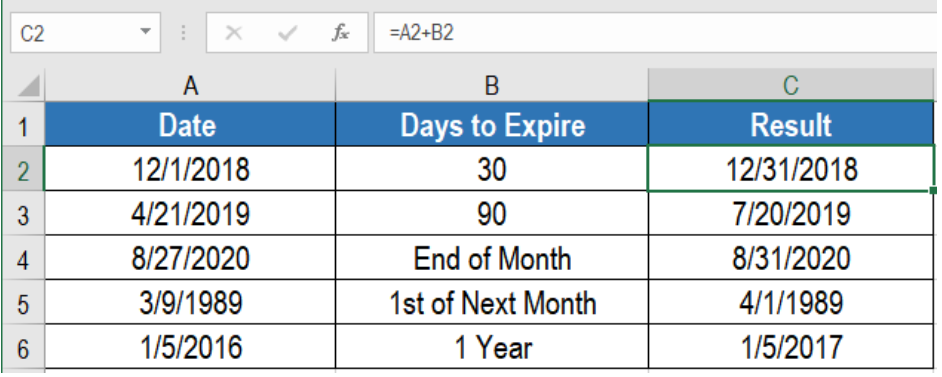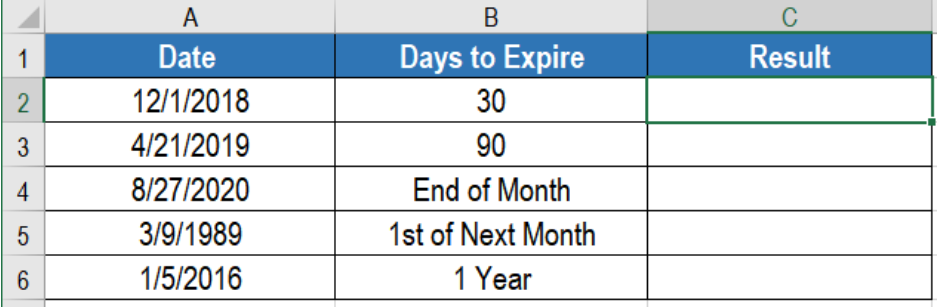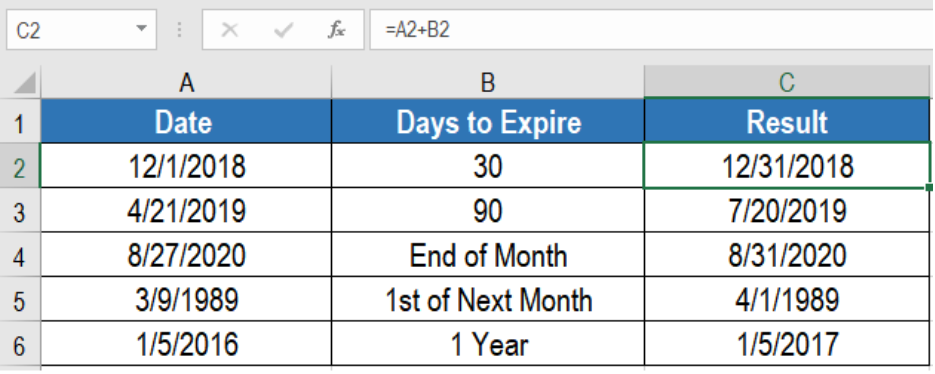Get instant live expert help with Excel or Google Sheets“My Excelchat expert helped me in less than 20 minutes, saving me what would have been 5 hours of work!”

#### Post your problem and you’ll get expert help in seconds.

Your message must be at least 40 characters
Our professional experts are available now. Your privacy is guaranteed.

# Calculate expiration date

Lookup is one of the mostly performed tasks in Microsoft Excel. We often require to perform lookups on different data. Sometimes, the result needs to be an entire row. The combination of the INDEX and MATCH functions allow us to lookup an entire row. In this tutorial, we will learn how to lookup an entire row in Excel.Figure 1. Example of How to Calculate Expiration Dates in Excel

## Generic Formula

`=Date+Days`

## Setting up Data

The following example contains some sample dates and days to expiration. Column A,and b has these dates and days.Figure 2. The Sample Data Set

## Process

We can use the following formulas to calculate the expiration dates in column C.Figure 3. Applying the formulas

#### `=A2+B2`

We use this for the dates in A2 and A3. The generic formula uses a simple addition of the days with the date given. We can use other functions to calculate the expiration date as well. Excel processes dates as serial numbers. According to this system, January 1, 1900 has the serial number 1. Continuing the numbers, January 1, 2050 is the serial number 54,789.

#### `=EOMONTH(A4,0)`

We can use the EOMONTH function to get the last day of the month. It returns the months in the future or past.

#### `=EOMONTH(A5,0)+1`

We can use the EOMONTH function to calculate the first day of the month as well. All we have to do is to use EOMONTH to get the last day of the previous month. Then we add 1 with it.

#### `=EDATE(A6,12)`

We can use the EDATE function to calculate expiration dates by months. EDATE returns the same date and months ahead or before.

This will show the expiration dates in column C.

Most of the time, the problem you will need to solve will be more complex than a simple application of a formula or function. If you want to save hours of research and frustration, try our live Excelchat service! Our Excel Experts are available 24/7 to answer any Excel question you may have. We guarantee a connection within 30 seconds and a customized solution within 20 minutes.

### Did this post not answer your question? Get a solution from connecting with the expert.Another blog reader asked this question today on Excelchat:
Solution examplesindex and match with duplicates. I need to use another column as a reference, so my return value has two match the value of two things for it to return
Solved by E. H. in 60 minsHello, I have a big spreadsheet in which I need to know how many patients came in each month based on provider. I am using the following formula but I still get the N/A error. =INDEX('No Show Appts Data'!D:E, MATCH(1, 'No Show Appts Data'!D:D='No Shows Data'!M2)*('No Shows Data'!E:E='No Shows Data'!N1),0)) No Show Appts Data is the name of the sheet where Column D is Month Year of date in question and Column E is the provider. No Shows Data is the sheet where I am making all the formula calculation where Column M is Month and Year and Column N is the provider in question and therefore M2 is the month in question and N1 the provider in question. How do I fix this error? Thanks
Solved by F. H. in 40 minsI need to find an INDEX function that will convert the Call Day (a number 1-7) to the actual weekday found in row 1 of the DayofWeek named range. I don't know if I'm supposed to use the MATCH function as well or not.
Solved by D. D. in 11 minsI have two columns with names and a third one with email addresses. For every name that matches, I need to copy the email address to an empty column next to the matching name. .
Solved by E. U. in 15 minshelp with INDEX(IndexArea,MATCH(\$M\$5,MatchRows,0),MATCH(\$M\$6,MatchColumns,0))
Solved by Z. Y. in 12 mins## Subscribe to Excelchat.coAnother blog reader asked this question today on Excelchat: